## ML Aggarwal Class 7 Solutions for ICSE Maths Chapter 6 Ratio and Proportion Ex 6.2

Question 1.
Which of the following statements are true?
(i) 2.5 : 1.5 :: 7.0 : 4.2
(ii) $$\frac { 1 }{ 2 } :\frac { 1 }{ 3 } =\frac { 1 }{ 3 } :\frac { 1 }{ 4 }$$
(iii) 24 men : 16 men = 33 horses : 22 horses.
Solution:
(i) 2.5 : 1.5 :: 7.0 : 4.2
Product of extremes = 2.5 × 4.2 = 10.50
Product of means = 1.5 × 7.0 = 10.50
By cross product rule
Product of extremes = Product of means
2.5 : 1.5 :: 7.0 : 4.2 is true statement
(ii) $$\frac { 1 }{ 2 } :\frac { 1 }{ 3 } =\frac { 1 }{ 3 } :\frac { 1 }{ 4 }$$
Product of extremes = $$\frac { 1 }{ 2 } \times \frac { 1 }{ 4 } =\frac { 1 }{ 8 }$$
Product of means = $$\frac { 1 }{ 3 } \times \frac { 1 }{ 3 } =\frac { 1 }{ 9 }$$
By cross product rule
Product of extremes ≠ Product of means
$$\frac { 1 }{ 2 } :\frac { 1 }{ 3 } =\frac { 1 }{ 3 } :\frac { 1 }{ 4 }$$ is not a true statement.
(in) 24 men : 16 men = 33 horses : 22 horses
Product of extremes = 24 × 22 = 528
Product of means = 16 × 33 = 528
By cross product rule
Product of extremes = Product of means
24 men : 16 men = 33 horses : 22 horses is a true statement.

Question 2.
Check whether the following numbers are in proportion or not:
(i) 18, 10, 9, 5
(ii) 3, 3$$\frac { 1 }{ 2 }$$, 4, 4$$\frac { 1 }{ 2 }$$
(iii) 0.1, 0.2, 0.3, 0.6
Solution:
(i) 18, 10, 9, 5
Product of extremes = 18 × 5 = 90
Product of means = 10 × 9 = 90
By cross product rule
Product of extremes = Product of means
The numbers 18, 10, 9, 5 are in proportion.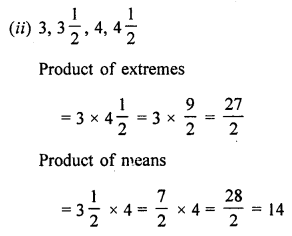By cross product rule
Product of extremes ≠ Product of means
The numbers 3, 3$$\frac { 1 }{ 2 }$$, 4, 4$$\frac { 1 }{ 2 }$$ are not in proportion.
(iii) 0.1, 0.2, 0.3, 0.6
Product of extremes = 0.1 × 0.6 = 0.06
Product of means = 0.2 × 0.3 = 0.06
By cross product rule
Product of extremes = Product of means
The numbers 0.1, 0.2, 0.3, 0.6 are in proportion.

Question 3.
Find x in the following proportions:
(i) x : 4 = 9 : 12
(ii) $$\frac { 1 }{ 13 }$$ : x :: $$\frac { 1 }{ 2 }$$ : $$\frac { 1 }{ 5 }$$
(iii) 3.6 : 0.4 = x : 0.5
Solution:
(i) x : 4 = 9 : 12
By cross product rule
Product of extremes = Product of means
x × 12 = 4 × 9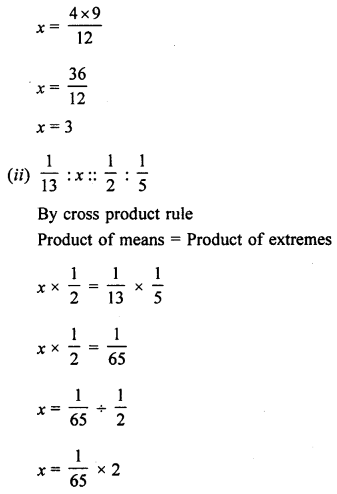Question 4.
Find the fourth proportional to
(i) 42, 12, 7
(ii) $$\frac { 1 }{ 3 }$$, $$\frac { 1 }{ 4 }$$, $$\frac { 1 }{ 5 }$$
(iii) 3 kg, 12 kg, 15 kg
Solution:
(i) 42, 12, 7
Let the fourth proportional be x.
Then 42, 12, 7, x are in proportion
Using the cross product rule
Product of extremes = Product of means
42 × x = 12 × 7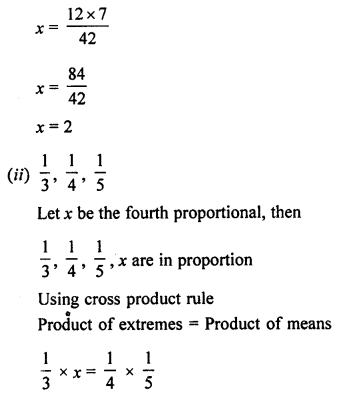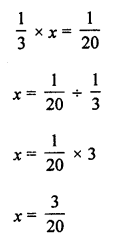(iii) 3 kg, 12 kg, 15 kg
Let the fourth proportional be x kg, then
3 kg, 12 kg, 15 kg, x kg are in proportion
Using cross product rule
Product of extremes = Product of means
3 × x = (12 × 15) kg
3x = 180 kg
x = 60 kg

Question 5.
Check whether 7, 49, 343 are in continued proportion or not.
Solution:
Three quantities are said to be in continued proportion if a : b = b : c i.e., if
$$\frac { a }{ b }$$ = $$\frac { b }{ c }$$ i.e., if b2 = ac
Here, a = 7, b = 49, c = 343
b2 = ac
(49)2 = 7 × 343
49 × 49 = 7 × 343
2401 = 2401
Yes, the number 7,49, 343 are in continued proportion.

Question 6.
Find the third proportional to
(i) 36, 18
(ii) 5$$\frac { 1 }{ 4 }$$, 7
(iii) 3.2, 0.8
Solution:
(i) 36, 18
Let the third proportional to 36, 18 be x.
Then 36, 18 and x are in continued proportion
36 : 18 :: 18 : x
Using the cross product rule
Product of extremes = Product of means
36 × x = 18 × 18
x = 9
Hence, the third proportion is 9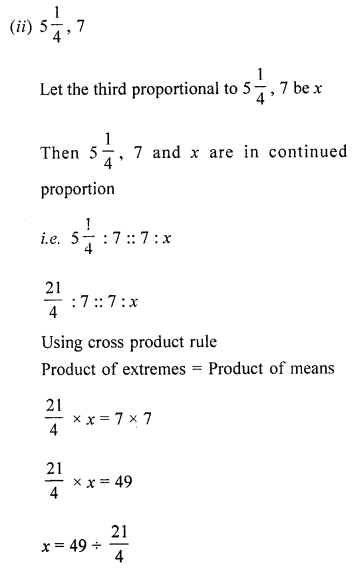(iii) 3.2, 0.8
Let the third proportional to 3.2, 0.8 be x.
Then 3.2, 0.8 and x are in continued proportion
i.e., 3.2 : 0.8 :: 0.8 : x
Products of extremes = 3.2 × x
Product of means = 0.8 × 0.8
3.2 × x = 0.8 × 8
x = 0.2
Hence, third proportion is 0.2

Question 7.
The ratio between the length and width of a rectangular sheet of paper is 7 : 5. If the width of the sheet is 20.5 cm, find its length.
Solution:
Let the length of the sheet be x.
Then the ratio of length to width is x : 20.5 cm.
According to given statement,
x : 20.5 cm = 7 : 5
Using cross product rule
Product of extremes = Product of means
x × 5 = 20.5 cm × 7
x × 5 = 143.5 cm
x = 28.7 cm
Hence, length of the sheet = 28.7 cm

Question 8.
The ages of Amit and Archana are in the ratio 4 : 5. If Amit is 4 years 8 months old, find the age of Archana.
Solution:
Let the age of Archana be x.
Then the ratio of ages of Amit and Archana be 4 years 8 months : x.
1 year = 12 months
4 years = 4 × 12 months = 48 months
4 years 8 months = (48 + 8) months = 56 months
According to given statement,
56 months : x :: 4 : 5
Using cross product rule
Product of means = Product of extremes
x × 4 = 56 months × 5
x = 70 months
Converting months in years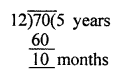70 months = 5 years 10 months
Hence, the age of Archana is 5 years 10 months.